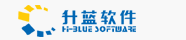# 没有定义变量的问题

March 25,2004

...
<Table>
...
</Table>
<%
IF Not IsNull(oRs("E_DT")) Then
%>
<Table>
...
</Table>
<%
End IF
%>

<%
Dim strBack
IF IsNull(oRs("E_DT")) Then
strBack = "0"
Else
strBack = "1"
End IF
%>
...
<Table>
...
</Table>
<%
IF strBack = "1" Then
%>
<Table>
...
</Table>
<%
End IF
%>